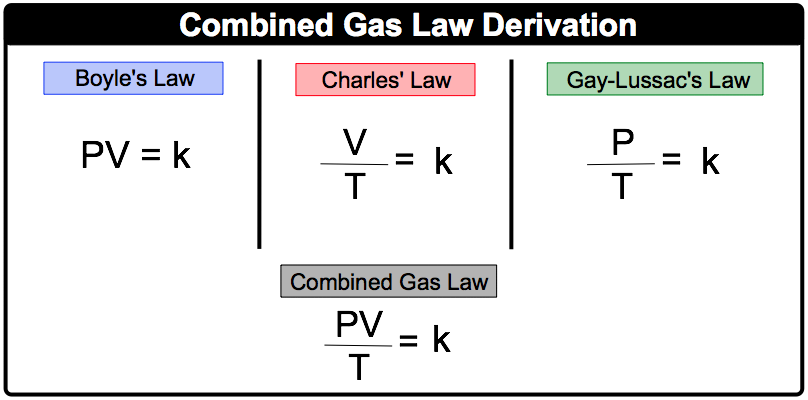Clutch Prep is now a part of Pearson

# Chemistry Gas Laws: Combined Gas Law

See all sections
Sections
Pressure Units
The Ideal Gas Law
The Ideal Gas Law Derivations
The Ideal Gas Law Applications
Chemistry Gas Laws
Chemistry Gas Laws: Combined Gas Law
Mole Fraction
Partial Pressure
The Ideal Gas Law: Molar Mass
The Ideal Gas Law: Density
Gas Stoichiometry
Standard Temperature and Pressure
Effusion
Root Mean Square Speed
Kinetic Energy of Gases
Maxwell-Boltzmann Distribution
Velocity Distributions
Kinetic Molecular Theory
Van der Waals Equation
Boyle's Law (IGNORE)
Charles Law (IGNORE)
Ideal Gas Law (IGNORE)

The Combined Gas Law is created from “combining” Boyle’s Law, Charles’ Law, and Gay–Lussac’s Law.

###### Combined Gas Law

Concept #1: The Combined Gas LawExample #1: A sample of gas initially has a volume of 900 mL at 520 K and 1.85 atm. What is the pressure of the gasif the volume decreases to 330 mL while the temperature increases to 770 K?

Practice: A 4.30 L gas has a pressure of 7.0 atm when the temperature is 60.0 ºC. What will be the temperature of the gas mixture if the volume and pressure are decreased to 2.45 L and 403.0 kPa respectively?

Practice: A sealed container with a movable piston contains a gas with a pressure of 1380 torr, a volume of 820 mL and a temperature of 31°C. What would the volume be if the new pressure is now 2.83 atm, while the temperature decreased to 25°C?